### Material Dispersion Evaluation

 Using Material Dispersion option it is possible to calculate the dispersion accumulated by a pulse passing through dispersive medium of the given thickness.   OptiLayer calculates phase ($$\varphi$$), group delay (GD) and group delay dispersion (GDD) accumulated in a substrate or in a stack (a set of thick media). $\varphi=\frac{\omega n(\omega)}{c}\cdot d_s,$ $GD=-\frac{d\varphi}{d\omega},$ $GDD=-\frac{d^2\varphi}{d^2\omega},$ $$d_s$$ is substrate thickness This option is available through Analysis > Material Dispersion You need to specify the type of medium (it is taken from the Substrate database) and its thickness (Fig. 1).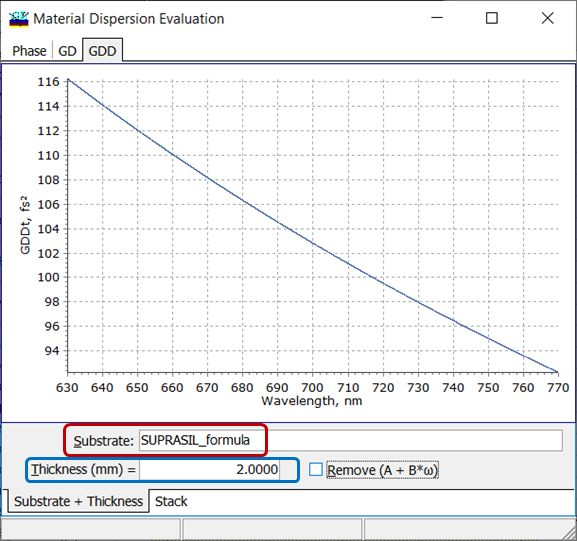Fig. 1. GDD accumulated in 2mm Suprasil. If a combination of several media need to be analyzed, it can be performed with the help of Stack object (Fig. 2). In this case all Media specified in Stack object are considered. Coatings (if they present) are ignored during Material Dispersion Evaluation.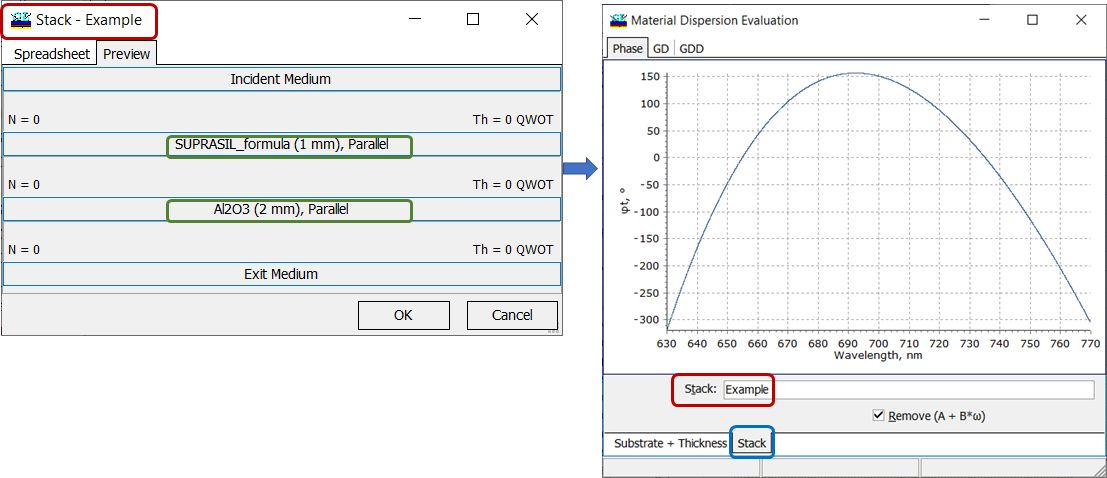Fig. 2. Phase accumulated in 1mm Suprasil and 2mm Sapphire. Optionally it is possible to exclude the term $$(A + B\omega)$$ from phase characteristic and constant term $$B$$ from GD plot. These terms don't affect the GDD characteristic (Fig. 3).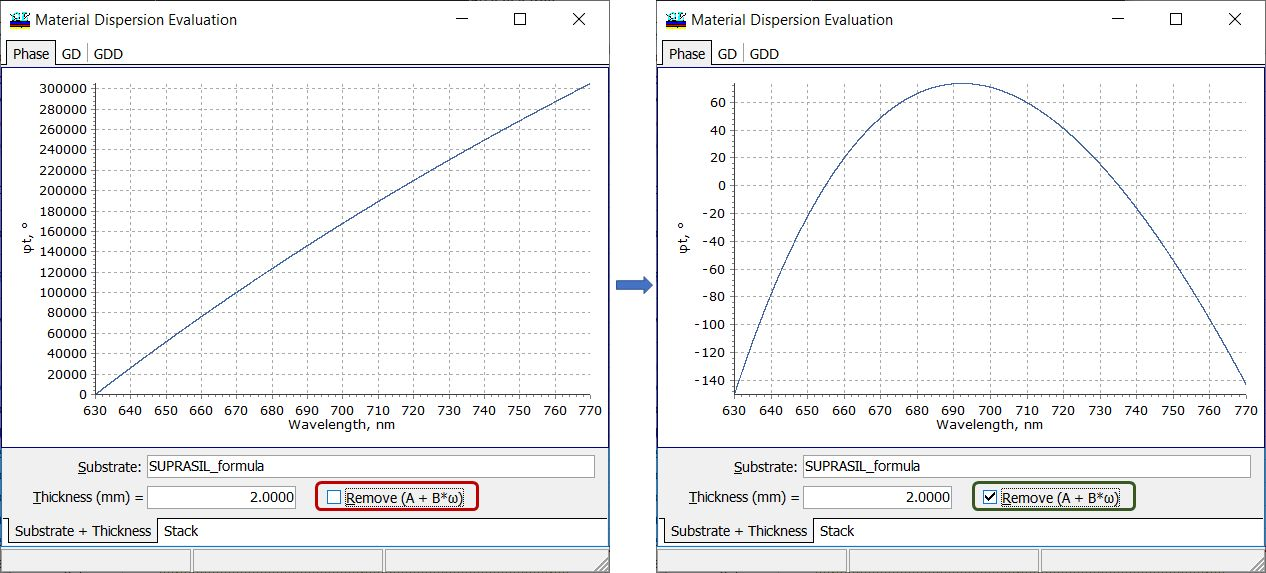Fig. 3. Phase accumulated in 2mm Suprasil with included $$(A + B\omega)$$ term (left panel) and excluded the linear term (right panel). In the case of stack (a set of media), accumulated phase, GD and GDD are calculated as a sum of phase, GD, and GDD accumulated in the corresponding media. For example, for the structure in Fig.   $\varphi(Stack)=\varphi(Suprasil)\cdot 1mm+\varphi(Sapphire)\cdot 2mm$ $GD(Stack)=GD(Suprasil)\cdot 1mm+GD(Sapphire)\cdot 2mm$ $GDD(Stack)=GDD(Suprasil)\cdot 1mm+GDD(Sapphire)\cdot 2mm$ You can obtain numerical values of the phase, GD, and GDD on a desired wavelength grid (Fig. 4).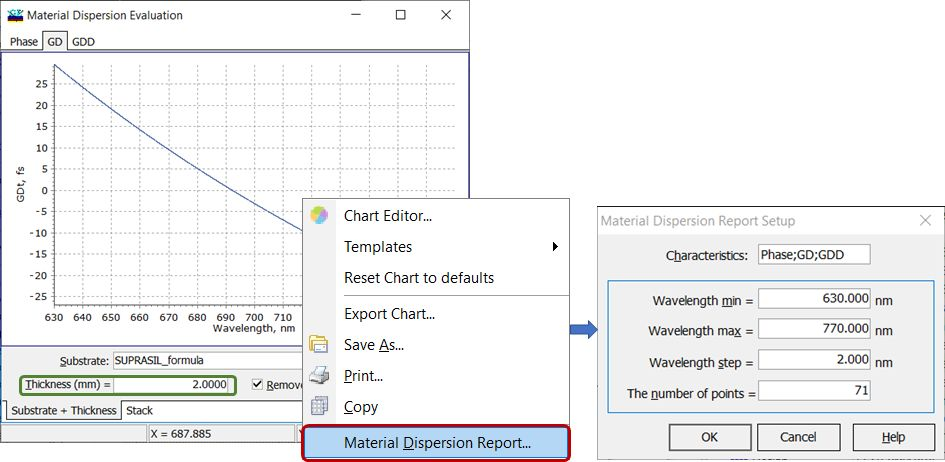Fig. 4. Numerical values of dispersion curves can be easily obtained through right-click menu or through Results--> Materials Dispersion Report. Important. Spectral range for calculations is the same as in Analysis window of OptiLayer. Important. Substrate object (Substrate + Thickness mode) or all Media in the Stack object (Stack mode) should be represented as formulas, since calculation of GD/GDD requires differentiation operations. GD/GDD values can be copied directly to design target. Important: in order to design a dispersive mirror compensating the accumulated dispersion, the sign of GD/GDD must be changed to the opposite one (Fig. 5).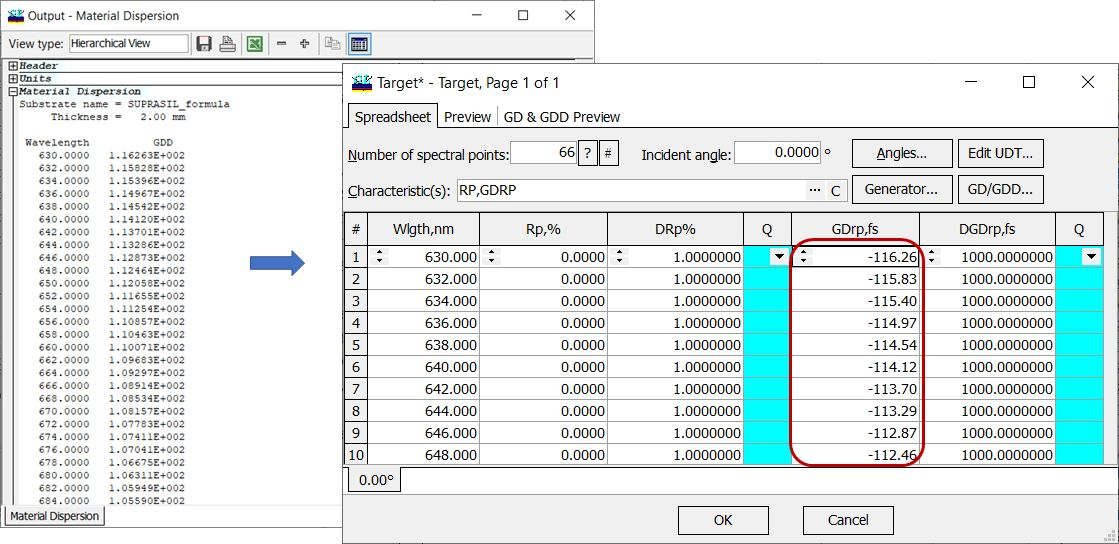Fig. 5. Numerical values of dispersion curves can be easily obtained through right-click menu or through Results--> Materials Dispersion Report. You may be interested to reading further articles: Coatings for laser applications Phase/GD/GDD targets

### Easy to startOptiLayer provides user-friendly interface and a variety of examples allowing even a beginner to effectively start to design and characterize optical coatings.        Read more...

### Docs / SupportComprehensive manual in PDF format and e-mail support help you at each step of your work with OptiLayer.If you are already an experienced user, OptiLayer gives your almost unlimited opportunities in solving all problems arising in design-production chain. Visit our publications page and challenge page.